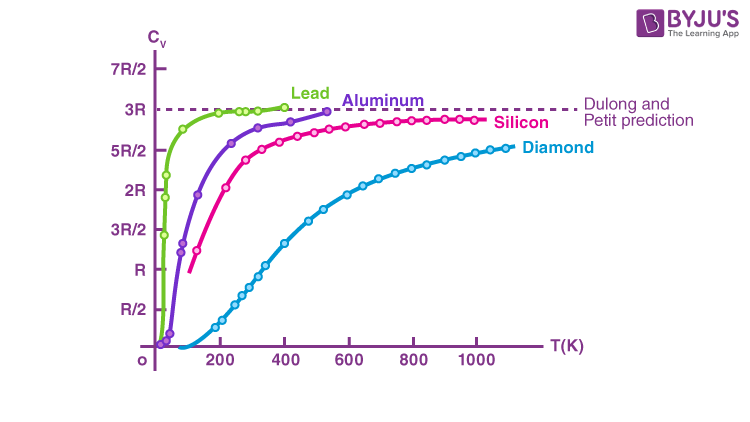# Dulong Petit Law

## What is the Dulong Petit Law?

The Dulong Petit Law states that the product of specific heat and atomic mass or gram-atomic heat capacity of an element is always a constant. It is constant for all solid elements.

The law was developed in 1819 on the grounds of examinations by Pierre-Louis Dulong and Alexis-Thérèse Petit. If the value of specific heat of the material is available, its atomic mass can be calculated using this fundamental law. Many atomic masses were derived. Later, it was altered to use only on metallic materials. It is now applied only as an estimation at intermediately large temperatures.

## Dulong Petit Law Equation

The Dulong Petit law is used to calculate the atomic mass and valency of elements.

The formula is,

$$\begin{array}{l}c \times M = k\end{array}$$

Where,

c = specific heat capacity

M = molar mass

k = constant

## Dulong Petit Law Relevances

Both Dulong and Petit were not aware of the ‘k’ constant. In the current era, this constant has become equivalent to 3R ( ‘R’, the gas constant).

So Dulong Petit law became:

$$\begin{array}{l}C \times M = 3R\end{array}$$

The number of moles is given by dividing mass m by molar mass M.

$$\begin{array}{l}C \times \frac{M}{m} = 3R \end{array}$$

$$\begin{array}{l}\frac{C}{n} = 3R\end{array}$$
Where,

C = heat capacity of the element

M = molar mass

m = mass of the material

n = number of moles

Classical thermodynamics laws are insignificant at low temperatures as quantum mechanics takes over the role. Therefore, the Dulong Petit Law also fails at temperatures below 120 K.

### Dulong Petit Prediction GraphThe law is applicable only at high temperatures.

In the above diagram, the curve of Silicon is flattening out after 600K, and the Lead curve is flattening after 200k. This graph clearly shows that the law is applicable at various higher temperatures for different elements.

### Limitations of Dulong Petit Law

• The Dulong Petit law is only relevant to the heavier elements.
• It is only applicable to elements that are in solid form.
• It cannot be applied to lighter elements having high melting points.
• It only gives a rough atomic mass.

## What Is Heat? Why Do We Experience It? How Does It Travel?### Dulong Petit Law Example

1) Find the atomic weight of material having a specific heat capacity equivalent to 600 J K-1kg-1

Answer: Specific heat capacity of the material

$$\begin{array}{l}C = 3\frac{R}{M}\end{array}$$
$$\begin{array}{l}\therefore M = 3\frac{R}{C}\end{array}$$
$$\begin{array}{l}M = 3 \times \frac{8.314}{600}\end{array}$$
$$\begin{array}{l}M = 0.0415 \cdot kg\cdot mole^{-1} = 40.15\cdot g \cdot mole^{-1}\end{array}$$

## Frequently Asked Questions – FAQs

Q1

### What is the Dulong Petit Law?

The Dulong Petit law states that the product of specific heat and atomic mass or gram-atomic heat capacity of an element is always a constant.
Q2

### What is the equation of the Dulong Petit Law?

$$\begin{array}{l}c \times M = k\end{array}$$

Where,

c = specific heat capacity

M = molar mass

k = constant

Q3

### What are the limitations of the Dulong Petit Law?

The Dulong Petit Law is only relevant to the heavier elements. It is only applicable to elements that are in solid form. It cannot be applied to lighter elements having high melting points. Finally, it only gives a rough atomic mass value.

Q4

### What is atomic mass?

It is the amount of matter inside an atom. It is measured in ‘unified atomic mass’ or dalton unit.

Q5

### Who developed the Dulong Petit Law?

The law was developed in 1819 on the grounds of examinations by Pierre-Louis Dulong and Alexis-Thérèse Petit.

Stay tuned to BYJU’S and Fall in Love with Learning!